Question

# write a java code to calculate a restaurant tip based on the diner’s satisfaction on a...

write a java code to calculate a restaurant tip based on the diner’s satisfaction on a scale of 1 – 5. The user will input the total bill and their rating. The program should output the tip percentage, tip amount, and total due. You must use a select statement instead of an if.
For a rating of 1, calculate a 10 % tip.
For a rating of 2, calculate a 12.5% tip.
For a rating of 3, calculate a 15 % tip.
For a rating of 4, calculate a 20 % tip.
For a rating of 5, calculate a 25 % tip.

```import java.util.Scanner;

public class RestaurantTip {
public static void main(String[] args) {
Scanner scan = new Scanner(System.in);
System.out.print("Enter bill: ");
double bill = scan.nextDouble();
System.out.print("Enter rating: ");
int rating = scan.nextInt();

double tip = 0.0;

switch (rating){
case 1:
System.out.println("Tip percentage: 10%");
tip = bill*0.10;
break;
case 2:
System.out.println("Tip percentage: 12.5%");
tip = bill*0.125;
break;
case 3:
System.out.println("Tip percentage: 15%");
tip = bill*0.15;
break;
case 4:
System.out.println("Tip percentage: 20%");
tip = bill*0.20;
break;
case 5:
System.out.println("Tip percentage: 25%");
tip = bill*0.25;
break;
}

System.out.println("Tip amount: "+tip);
System.out.println("Total due: "+(bill+tip));
}
}
```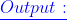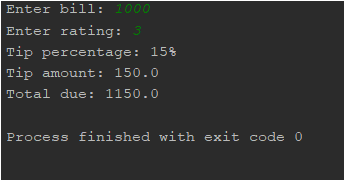#### Earn Coins

Coins can be redeemed for fabulous gifts.

Similar Homework Help Questions
• ### 1. Need help with a Java application that will compute tip amounts based on user input....

1. Need help with a Java application that will compute tip amounts based on user input. Please let me know what else my code could use. My code is below and I need help formatting the output and calculating the amount paid by each person. Your application will: ask the user for a restaurant name, a waiter/waitress name, a bill amount and the number of people splitting the bill calculate 10%, 15% and 20% tips on the bill amount calculate...

• ### in C# Write an object-oriented program that can be used to determine the tip amount that...

in C# Write an object-oriented program that can be used to determine the tip amount that should be added to a restaurant charge. Allow the user to input the total, before the taxes (9% of the total) and the tip charge of 15%. Produce output showing the calculated total amount due. Tax should be added to the bill before the tip is determined. i will rate if correct

• ### Question 4 (3 mark) : Write a Java program to ask the user to input an...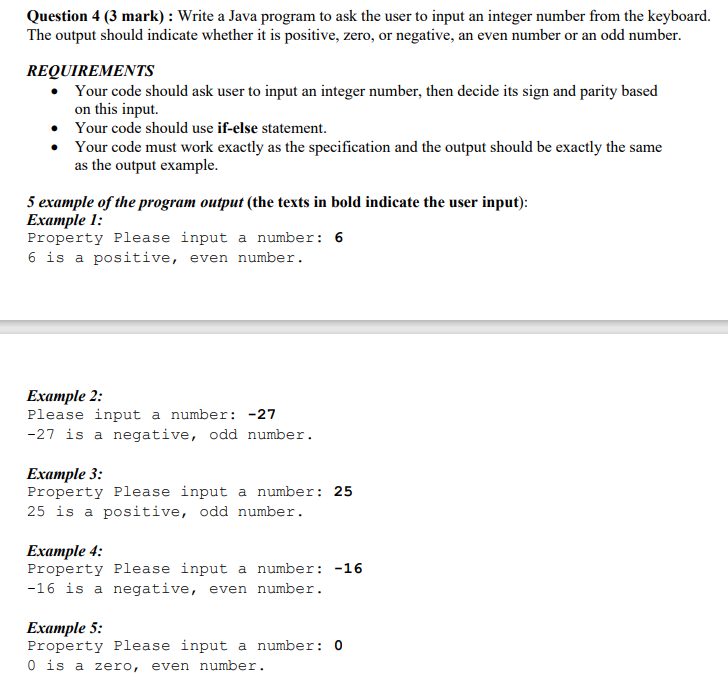Question 4 (3 mark) : Write a Java program to ask the user to input an integer number from the keyboard. The output should indicate whether it is positive, zero, or negative, an even number or an odd number. REQUIREMENTS • Your code should ask user to input an integer number, then decide its sign and parity based on this input. • Your code should use if-else statement. Your code must work exactly as the specification and the output should...

• ### Write a C  program that computes the tax and tip on a restaurant bill for a patron...

Write a C  program that computes the tax and tip on a restaurant bill for a patron with a \$88.67 meal charge. The tax should be 6.75 percent of the meal cost. The tip should be 20 percent of the total after adding the tax. Display the meal cost, tax amount, tip amount, and total bill on the screen.

• ### Write a java code based on the below question. Q4 revision: Assume the following is ur...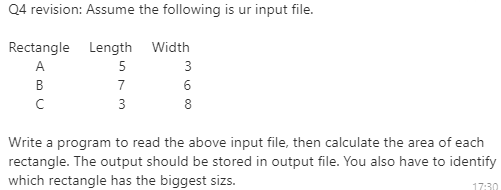Write a java code based on the below question. Q4 revision: Assume the following is ur input file. 5 Rectangle Length Width A 3 B 7 6 с 3 8 Write a program to read the above input file, then calculate the area of each rectangle. The output should be stored in output file. You also have to identify which rectangle has the biggest sizs. 17:30

• ### Java programming Create a simple tip calculator called TipCalcMethod. Your program should read in the bill...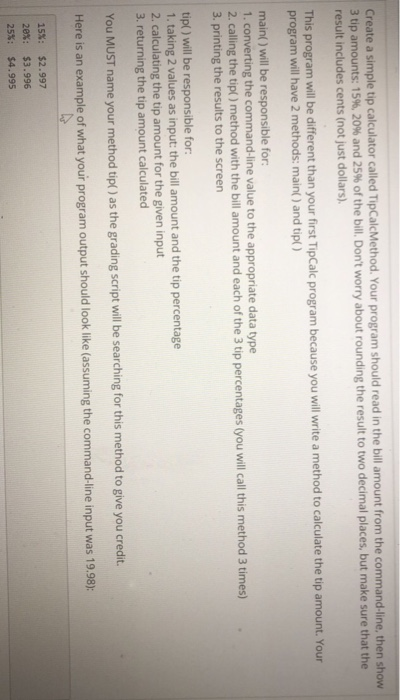Java programming Create a simple tip calculator called TipCalcMethod. Your program should read in the bill amount from the command-line, then show 3 tip amounts: 15%, 20% and 25% of the bill. Don't worry about rounding the result to two decimal places, but make sure that the result includes cents (not just dollars). This program will be different than your first Tipcalc program because you will writea method to calculate the tip amount. program will have 2 methods: main) and...

• ### write a program to calculate the amount of a server's tip given the amount of the bill and the percentage tip ovtained via input dialog boxes

write a program to calculate the amount of a server's tip given the amount of the bill and the percentage tip ovtained via input dialog boxes. The output should be a complete sentence that reiterates the inputs and gives the resulting tip. here is my attempt:Public Class frmTip Private Sub btnEnter_Click(ByVal sender As System.Object, ByVal e As System.EventArgs) Handles btnEnter.Click Dim billAmount As Double Dim percent, tip As Double billAmount = CDbl(InputBox("Amount of Bill")) percent = CDbl(InputBox("Amount of Tip")) tip...

• ### Python Simple Programming Write a program in Python that calculates the tip and total for a...

Python Simple Programming Write a program in Python that calculates the tip and total for a meal at a restaurant. When your program is run it should ... Calculate the tip and total for a meal for a restaurant Print the name of the application "Tip Calculator" Get input from the user for cost of meal and tip percent Print the tip and total amounts. The formula for calculating the tip amount is: tip = cost of meal * (tip...

• ### Homework description::::: Write JAVA program with following description. Sample output with code will be helful... A...

Homework description::::: Write JAVA program with following description. Sample output with code will be helful... A compiler must examine tokens in a program and decide whether they are reserved words in the Java language, or identifiers defined by the user. Design a program that reads a Java program and makes a list of all the identifiers along with the number of occurrences of each identifier in the source code. To do this, you should make use of a dictionary. The...

• ### In C++ Programming Write a program in to help a local restaurant automate its breakfast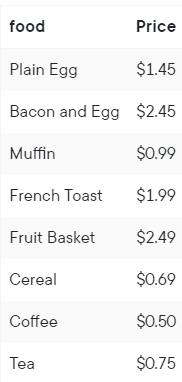Write a program to help a local restaurant automate its breakfast billing system. The program should do the following:Show the customer the different breakfast items offered by the restaurant.Allow the customer to select more than one item from the menu.Calculate and print the bill.Assume that the restaurant offers the following breakfast items (the price of each item is shown to the right of the item):Use an array menuList of type menuItemType, as defined in Programming Exercise 3. Your program must contain at least the...